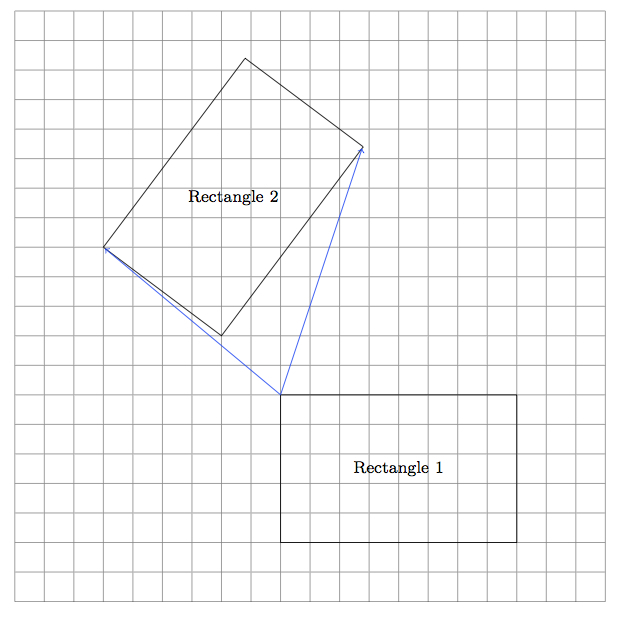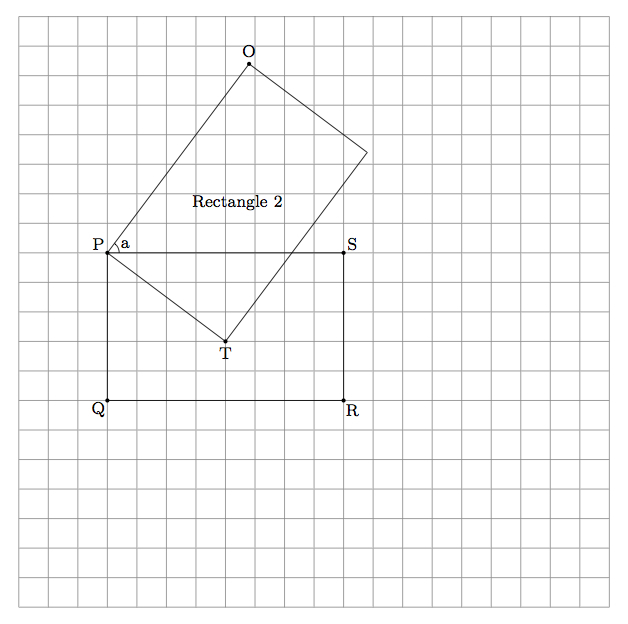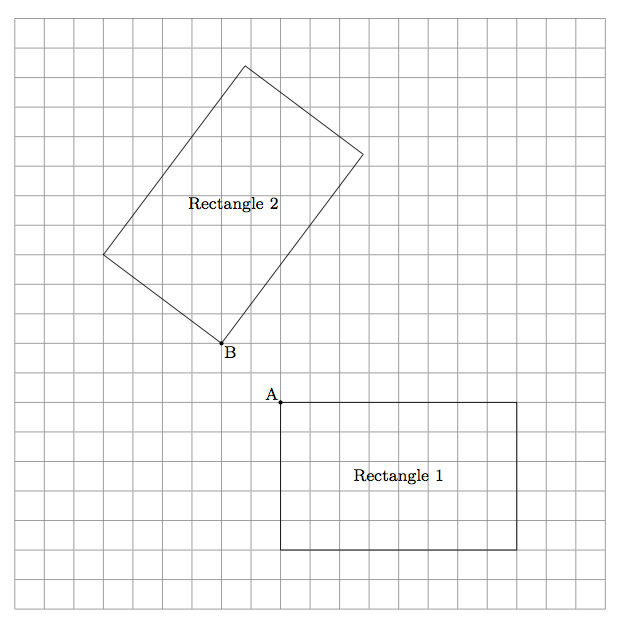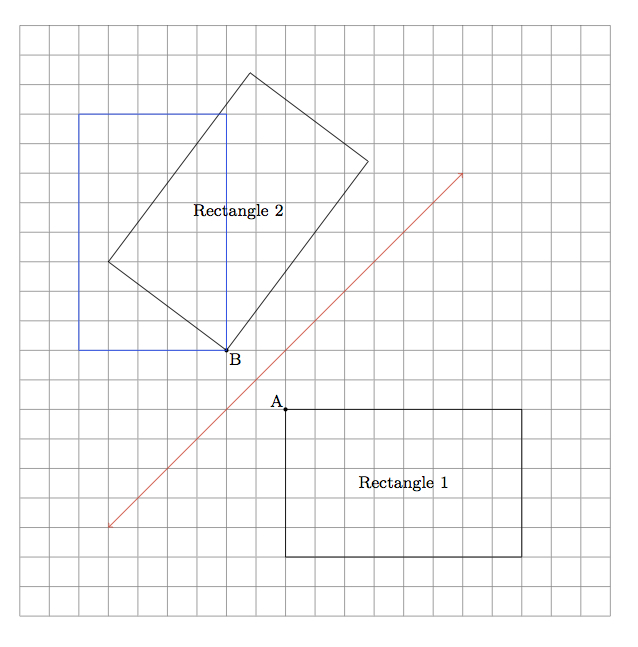# Congruent Rectangles

Alignments to Content Standards: 8.G.A.2

Below is a picture of two rectangles with the same length and width:1. Show that the rectangles are congruent by finding a translation followed by a rotation which maps one of the rectangles to the other.
2. Explain why the congruence of the two rectangles can not be shown by translating Rectangle 1 to Rectangle 2.
3. Can the congruence of the two rectangles be shown with a single reflection? Explain.

## IM Commentary

This task is designed to give students insight into the effects of translations, rotations, and reflections on geometric figures in the context of showing that two figures are congruent. Since the two rectangles are congruent, there must be some sequence of translations, rotations, and reflections which will take Rectangle 1 to Rectangle 2 (and vice versa). In practice, there are many possible ways to show a congruence and this should be emphasized in part (a). Students might be encouraged to work on their own to find a congruence before working on the three parts of this problem. Instead of a translation followed by a rotation, a rotation followed by a translation is also a natural choice. Or the rotation could be replaced with an appropriate reflection (this is more challenging).

Parts (b) and (c) of the problem show that while there is substantial flexibility in choosing rigid motions to show a congruence, there are some limitations. Part (c) requires good geometric intuition and perhaps some experimentation with reflections. The task is most appropriate for instructional purposes.

The figure provided in the stem of the task does not label the vertices of the rectangles, which provides students with an opportunity to choose to label them on their own, allowing them to engage in standard for mathematical practice 6, Attend to Precision. To see an in-depth look at how a teacher might guide such a discussion, take a look at the LessonSketch slideshow linked to our illustration of MP 6: Which Angles are Congruent?

## Solution

1. We can do this by choosing a vertex of Rectangle 1 and then translate this to a carefully selected vertex of Rectangle 2 and then follow this with a rotation about the chosen vertex of Rectangle 2. Pictured below are two possibilities for the translation:Below is the impact of translating by the lower left arrow which moves Rectangle 1 six boxes to the left and five boxes up to what is now labelled rectangle $PQRS$.:Also labelled in the picture are vertices $O$ and $T$ of Rectangle 2 and the angle measure $a = m(\angle OPS)$. Rotating rectangle $PQRS$ by angle $a$, counterclockwise, will bring ray $\overrightarrow{PS}$ to ray $\overrightarrow{PO}$. Since $\overline{PO}$ is congruent to $\overline{PS}$ this means that the rotation maps $S$ to $O$. We know that $m(\angle SPQ) = 90^\circ$ and $m(\angle OPT) = 90^\circ$. This means that the rotation by $a$ counterclockwise maps ray $\overrightarrow{PQ}$ to $\overrightarrow{PT}$. Since segments $\overline{PQ}$ and $\overline{PT}$ are congruent, this means that $Q$ maps to $T$. The three vertices $O,P,T$ determine Rectangle 2 so this translation followed by a rotation maps Rectangle 1 to Rectangle 2.

2. A translation can move Rectangle 1 to any other position on the coordinate grid but will not influence the angles its sides make with the grid lines. The lines containing the upper and lower sides of the rectangle will always be parallel to the horizontal lines of the grid while the lines containing the left and right sides of Rectangle 1 will always be parallel to the vertical lines in the grid. Since none of the lines containing sides of Rectangle 2 are parallel to the grid lines, Rectangle 1 cannot be moved to Rectangle 2 with a translation.

3. The congruence cannot be exhibited with a single reflection. To see this, we can choose a vertex of Rectangle 1. This is done in the picture below where the chosen vertex is denoted by $A$:A congruence of two rectangles maps vertices to vertices so this means that $A$ would have to map to one of the four vertices of Rectangle 2. Of these four reflections the one which maps Rectangle 1 closest to Rectangle 2 is the one which sends $A$ to $B$. Below is a picture, in red, of the line of reflection which interchanges $A$ and $B$ (the perpendicular bisector of segment $\overline{AB}$) and the image of Rectangle 1 under this reflection, in blue.Note that folding over a line which makes a $45^\circ$ angle with the horizontal and vertical grid lines interchanges the horizontal and vertical lines of the grid.

Similarly, any reflection that maps vertex $A$ to any of the other four vertices will not work either. So there is no reflection that maps one of the rectangles to the other.